Ex 12.1

Chapter 12 Class 9 Statistics
Serial order wise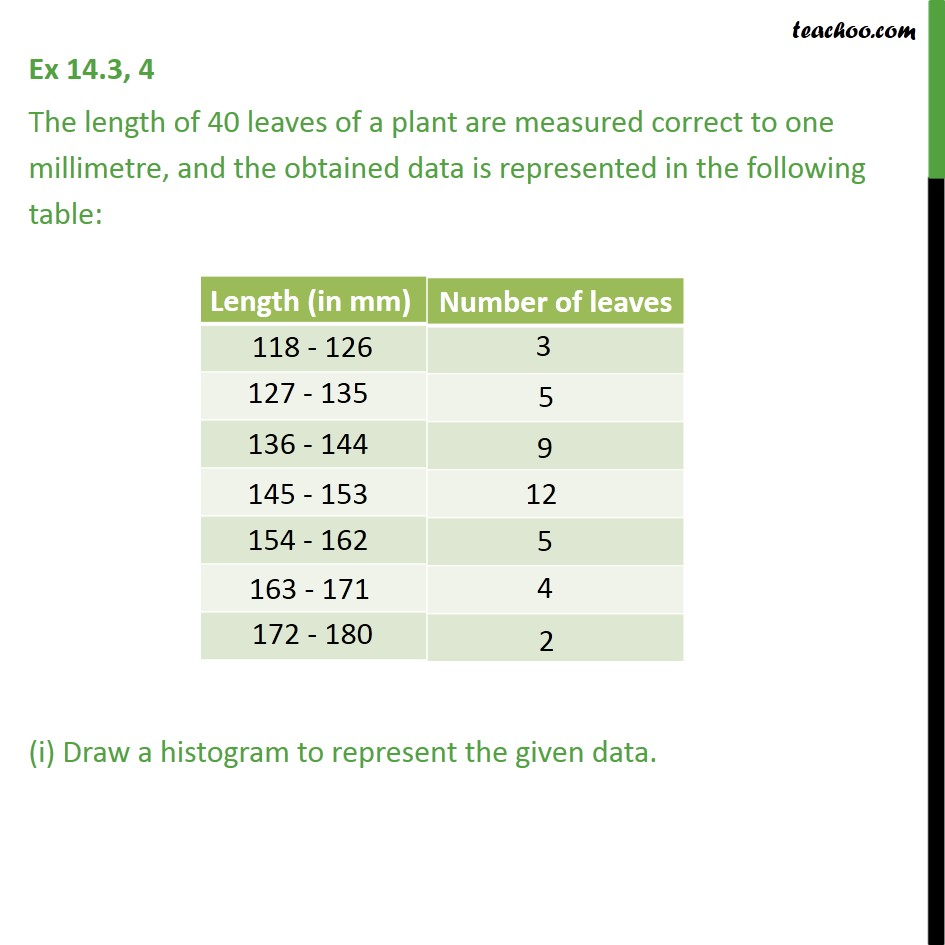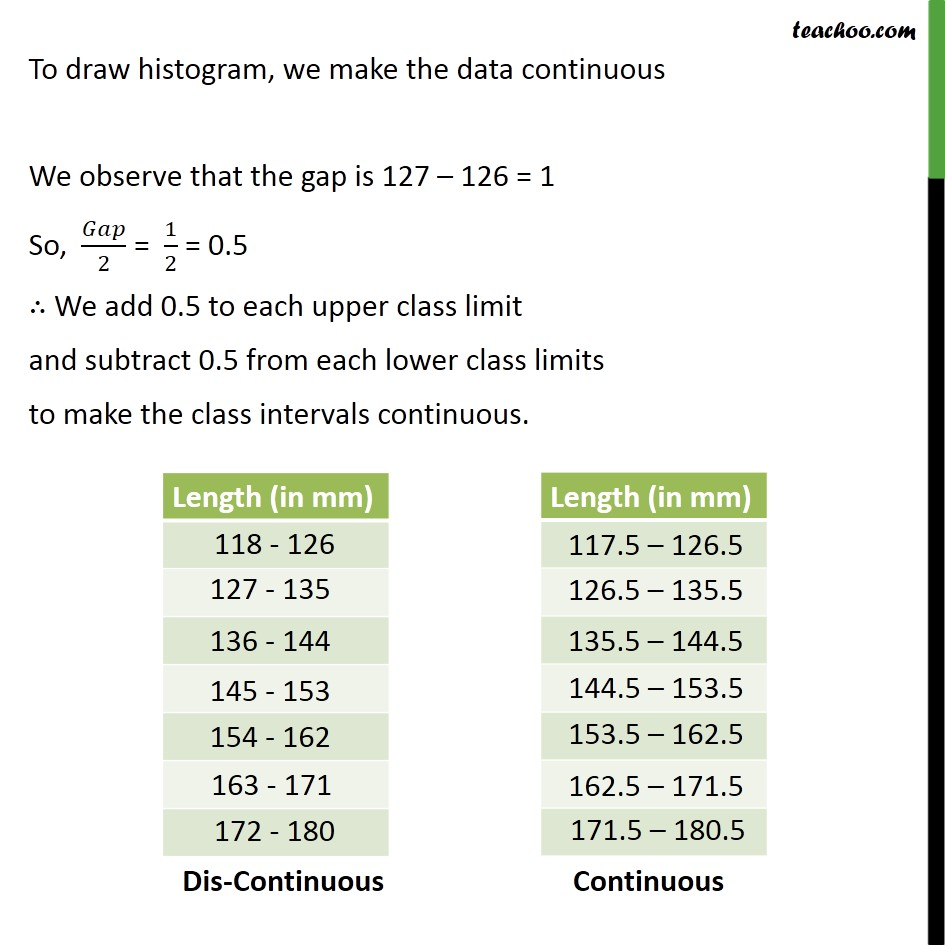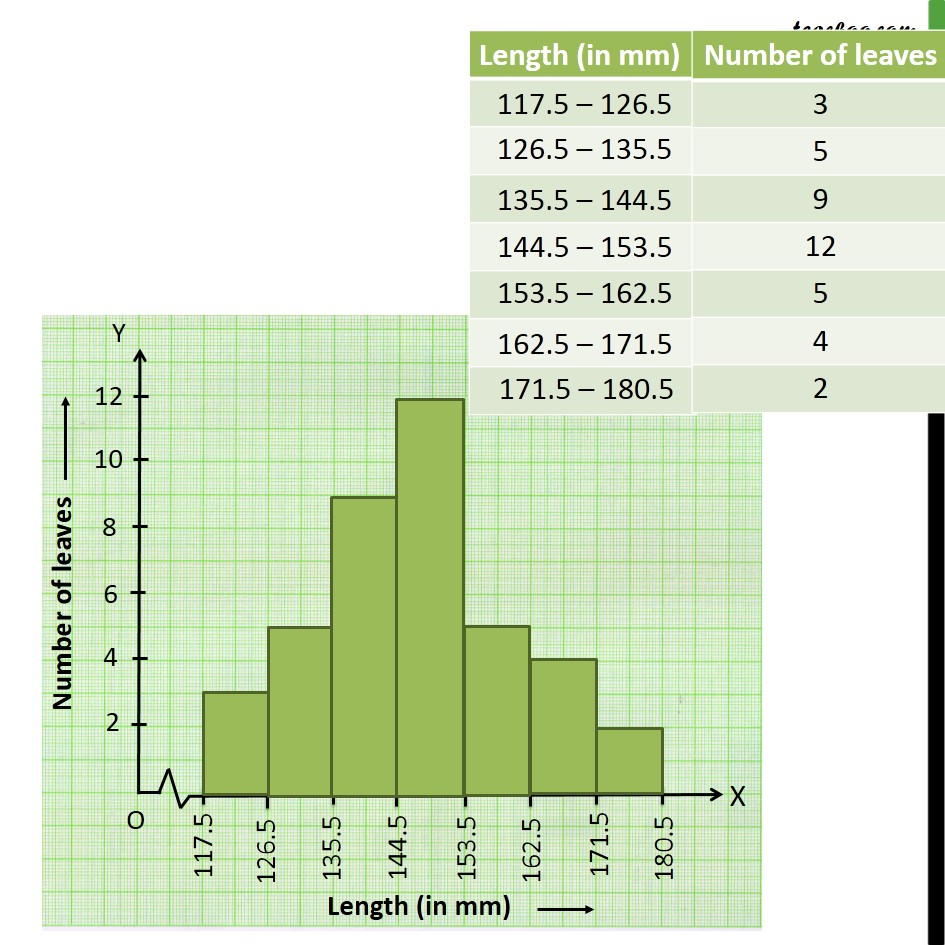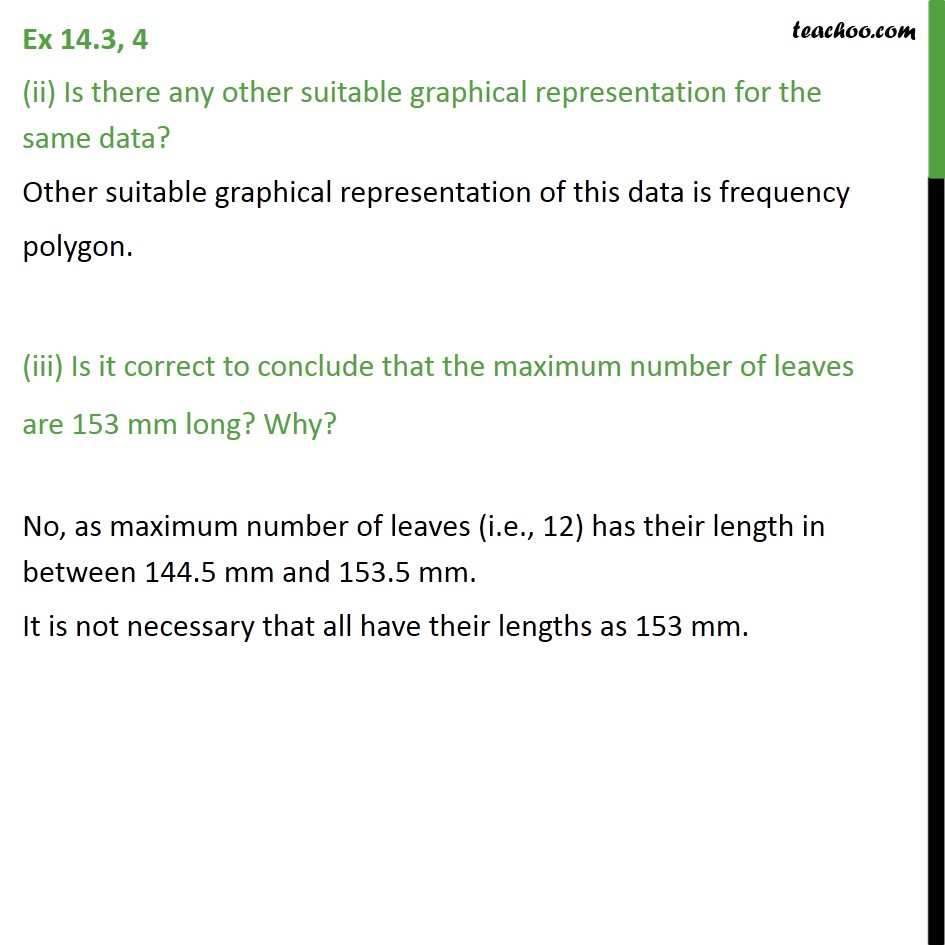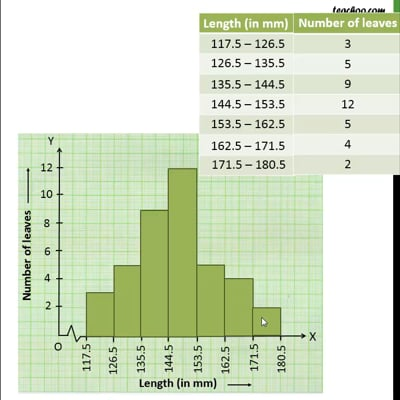This video is only available for Teachoo black users

Learn in your speed, with individual attention - Teachoo Maths 1-on-1 Class

### Transcript

Ex 12.1, 4 The length of 40 leaves of a plant are measured correct to one millimetre, and the obtained data is represented in the following table: (i) Draw a histogram to represent the given data. To draw histogram, we make the data continuous We observe that the gap is 127 – 126 = 1 So, 𝐺𝑎𝑝/2 = 1/2 = 0.5 ∴ We add 0.5 to each upper class limit and subtract 0.5 from each lower class limits to make the class intervals continuous. Ex 12.1, 4 (ii) Is there any other suitable graphical representation for the same data? Other suitable graphical representation of this data is frequency polygon. (iii) Is it correct to conclude that the maximum number of leaves are 153 mm long? Why? No, as maximum number of leaves (i.e., 12) has their length in between 144.5 mm and 153.5 mm. It is not necessary that all have their lengths as 153 mm.